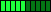Search    VB VB.net C# ASP.net ASP SQL Server VC++ My Links Networking ORACLE MySQL PHP Unix/Linux WinNT/2k/2003 Flash Maya 3D max Photoshop DB2 C/C++/ASM Microcontroller Circuit Design JAVA Wireless/Mobile JScript     Articles from this site only Links only Articles with downloads Articles with screenshot(s)  Code/Page 10 20 30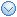User Login Email Password Remember Me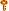Forgot the Password?Services
» Web Development
» Maintenance
» Data Integration/BI
» Information ManagementProgrammingVB (1648)VB.net (736)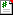C# (15)ASP.net (779)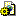ASP (41)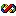VC++ (25)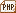PHP (0)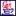JAVA (4)JScript (5)Database
 » SQL Server (708) » ORACLE (5) » MySQL (0) » DB2 (0)Automation
 » C/C++/ASM (11) » Microcontroller (1) » Circuit Design (0)OS/Networking
 » Networking (5) » Unix/Linux (1) » WinNT/2k/2003 (8)Graphics
 » Flash (0) » Maya (0) » 3D max (0) » Photoshop (0)Links
 » ASP.net (2) » PC Interfacing (1) » Networking (4) » SQL Server (4) » VB (23) » VB.net (4) » VC (3) » HTML/CSS/JavaScript (10)Tools
» Regular Expr Tester
» Free ToolsConvert a binary, octal, or hexadecimal value to decimal Total Hit (3018) The Convert class offers a few static methods that let you easily and quickly convert a binary, octal, or hexadecimal number(stored in a String) into the equivalent Byte, Short, Integer, or Long value. These are the methods in question: «Code LangId=2»Dim b As Byte = Convert.ToByte(value, fromB ....Read More Rating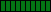Convert a decimal value to binary, octal, or hexadecimal Total Hit (4612) The ToString method of the Convert class lets you easily and quickly convert a decimal value into a string representation of that number to binary, octal, or hexadecimal base: «Code LangId=2»' convert to binary Console.WriteLine(Convert.ToString(11, 2)) ' => 1011 ' convert to octal Console.W ....Read More Rating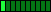MK? And CV? - Convert numbers to strings and back Total Hit (7581) The following routines convert a numeric value into a string that represents the number, and vice versa. They are useful for reading data written by QuickBasic programs, because the QuickBasic language functions that did the conversions were not ported to Visual Basic. Points to note: 1. These ....Read More RatingTake advantage of the new math functions Total Hit (1995) The System.Math class exposes several static methods that let you perform many common operations. These functions replace the VB6 functions with same name, but there are a few new functions that have no direct VB6 counterpart: Ceiling(x) returns the integer equal or higher than the argument Floo ....Read More Rating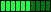Any2Dec - Convert from any numeric base to decimal Total Hit (1953) «Code LangId=2»' convert from any base to decimal ' BASE can be in the range 2-36 Function Any2Dec(ByVal otherBaseNumber As String, ByVal base As Integer) As Long Dim digits As String Dim digitValue As Long ' check base If base < 2 Or base > 36 Then Throw New Argum ....Read More RatingBin - Convert from decimal to binary Total Hit (2856) «Code LangId=2»' convert from decimal to binary ' if you pass the Digits argument, the result is truncated to that number of ' digits ' ' you should always specify Digits if passing negative values Function Bin(ByVal value As Long, Optional ByVal digits As Short = -1) As String ' conver ....Read More RatingBinToDec - Convert from binary to decimal Total Hit (2783) «Code LangId=2»' convert from binary to decimal Function BinToDec(ByVal value As String) As Long ' we just need a call to the Convert.ToInt64 static method Return Convert.ToInt64(value, 2) End Function «/Code» RatingDec2Any - Convert a decimal number to any other base Total Hit (3003) «Code LangId=2»' convert a positive number to any base ' BASE can be in the range 2-36 Function Dec2Any(ByVal number As Long, ByVal base As Short) As String Dim index As Integer Dim digitValue As Integer Dim res As New System.Text.StringBuilder() Const digits As String = "0 ....Read More RatingEvaluateModule - a module for evaluating expressions Total Hit (1703) «Code LangId=2»' A module for evaluating expressions, with support for ' parenthesis and many math functions ' Example: ' Dim expr As String = "(SQR(9)^3)+COS(0)*3+ABS(-10)" ' txtResult.Text = Evaluate(expr).ToString ' ==> 27+3+10 ==> 40 Imports System.Text.RegularExpressions M ....Read More RatingHex - Convert from decimal to hexadecimal Total Hit (2907) «Code LangId=2»' convert from decimal to hexadecimal ' if you pass the Digits argument, the result is truncated to that number of ' digits ' ' you should always specify Digits if passing negative values Function Hex(ByVal value As Long, Optional ByVal digits As Short = -1) As String ' c ....Read More RatingHex2Dec - Convert from hexadecimal to decimal Total Hit (6590) «Code LangId=2»' convert from hexadecimal to decimal Function HexToDec(ByVal value As String) As Long ' we just need a call to the Convert.ToInt64 static method Return Convert.ToInt64(value, 16) End Function «/Code» Rating